0
1913

# SSC Stenographer Reasoning Direction Questions PDF:

Download Reasoning Direction Questions For SSC Stenographer PDF. Practice Important Reasoning Directions sense test steno Questions and Answers based on asked questions in previous papers.

Question 1: Srishanth starts from his house and travels 6 km towards the north and takes a left turn to travel for 10.5 km. Then he takes a right turn to travel for another 3 km, finally he takes another right turn and travels for 3.8 km. Find the final direction Srishanth is facing to?

a) North

b) South

c) East

d) West

Question 2: Ram starts from his house and moves 26 km in North-West direction. He then takes a right turn and moves 7 km. Finally, he takes yet another right turn and moves another 2 km and stops at that point. What is the direction he is finally facing and the shortest distance between his final and starting point.

a) North-West, 25 km

b) South-East, 25 km

c) South-West, 22 km

d) None of these

Question 3: If you are facing west and travel by 100 m and then take a left and travel by 200 m, then in which direction are you facing?

a) east

b) west

c) north

d) south

Question 4: Ajay starts from his home and heads east. He walks for 3 km and then takes a left turn. After walking for another 2 km, he takes a right turn. He walks for another 3 km and then takes another left. He walks for 2 more km before coming to a halt. What is the shortest distance between his current position and his home?

a) 7 km

b) $2\sqrt{13}$

c) 10 km

d) $4\sqrt{5}$

Question 5: If a person is facing West and moves 15 m forward, turn left and move 15 m, then the person is

a) South of his initial position

b) South-West of his initial position

c) North-West of his initial position

d) West of his initial position

Question 6: B is 8 km to the west of A. C is 15 km to the north of B. What is the shortest distance between A and C?

a) 17 km

b) 19 km

c) 21 km

d) 23 km

Question 7: Dharam started from his home and started walking in the East direction. After walking for 5 km, he took a right turn and walked for 8 km. The then takes a left turn and walked for 3 km. Finally, he takes yet another left turn and walked for 2 km and stopped at that point.
What is the minimum distance between his starting and his final position and the direction he is finally facing?

a) North, 10 km

b) West, 10 km

c) South, 12 km

d) North, 12 km

Question 8: Kranthi starts from his house and travels 15 km towards the east and then takes a left turn to travel for another 20 km. Now, finally he takes a right turn and travels for 6 km to reach his destination. Find the shortest distance between his starting and ending points.

a) 33 km

b) 39 km

c) 29 km

d) 31 km

Question 9: Lohit walks 10m backward facing north and then, turns to his right to walk 10m forward. He then turns to his right and walks backward. In which direction is he walking now?

a) East

b) North

c) West

d) South

Question 10: Mohan starts from his home and heads north. He walks for 3 km and then takes a left turn. He walks for another 2 km and then takes a right turn. He walks for 1 km and then takes a left turn. He walks for another 1 km before stopping. What is the shortest distance between his current position and his initial position?

a) $3\sqrt{5}$ km

b) $4\sqrt{3}$ km

c) 5 km

d) 7 km

Question 11: Pankaj starts from his home and heads west. He walks for 4 km and then takes a right turn. He walks for another 3 km and then takes a left turn. He walks for another 2 km and then takes a right turn. He stops after walking for another 3 km. What is the shortest distance between his current position and his home?

a) $5\sqrt{3}$

b) 7 km

c) $6\sqrt{2}$ km

d) 9 km

Question 12: Ram starts moving towards north from a point A and takes a right turn at point B and moves forward to reach point C. He then takes a right turn and reaches point D. If AB = BC = CD, in which direction is point B from his current location?

a) North-East

b) North-West

c) South- East

d) South-West

Question 13: If Manoj starts running from a point towards North and after covering 4 km he turns to left and runs 5 km, and then again turn to left and runs 5 km. and then turn to left again and run another 6 km and before finishing he takes another left turn and runs 1 km. From the finishing point if he has to reach the point from where he started, in which direction will he has to run?

a) East

b) West

c) North

d) South

Question 14: If you are facing south and travel by 50 m and take a left and travel by 75 m and take a right, then in which direction will you be facing?

a) North

b) South

c) East

d) West

Question 15: Abhay travelled 9 km Northwards, turned left and travelled 5 km, then turned left again and travelled 9 km. How far is Abhay from the starting point?

a) 5 km.

b) 9 km.

c) 4 km.

d) 14 km.

Question 16: Sunita rode her scooty northwards, then turned left and then again rode to her left 4 km. She found herself exactly 2 kms West of her starting point. How far did she ride northwards initially?

a) 6 km

b) 2 km

c) 4 km

d) 5 km

Question 17: Pinky walks a distance of 600 metre towards East, turns left and moves 500 metre, then turns left and walks 600 metre and then turns left again and moves 500 metre and halts. At what distance in metres is she from the starting point?

a) 600

b) 2200

c) 500

d) 0

Question 18: If you start running from a point towards North and after covering 4 kms. you turn to your left and run 5 kms. and then again turn to your left and run 5 kms. and then turn to left again and run another 6 kms and before finishing you take another left turn and run 1 km., then answer the following question based on this information. From the finishing point if you have to reach the point from where you started, in which direction will you have to run ?

a) West

b) East

c) South

d) North

Question 19: Sohan drove 15 kms. to the west from his house, then turned left and walked 20 kms. He then turned East and walked 25 kms. and finely turning left covered 20 kms. How far he is from his house?

a) 5 Kms.

b) 40 Kms.

c) 10 Kms.

d) 80 Kms.

Question 20: Anand travels 10 kms from his home to the east to reach his school. Then he travels 5 kms to the south to reach his father’s shop, after school. He then travels 10 kms to the west to help his uncle. How far and in which direction is he from his home ?

a) 10 kms North

b) 5 kms South

c) 5 kms East

d) 10 kms West

Based on the given information, the following diagram could be derived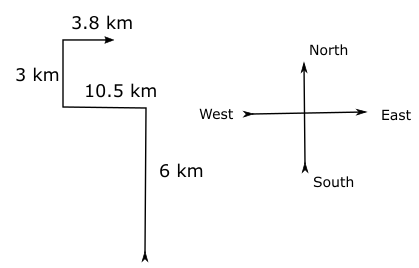According to the above diagram, the direction Srishanth finally facing is East.

Consider the following figure: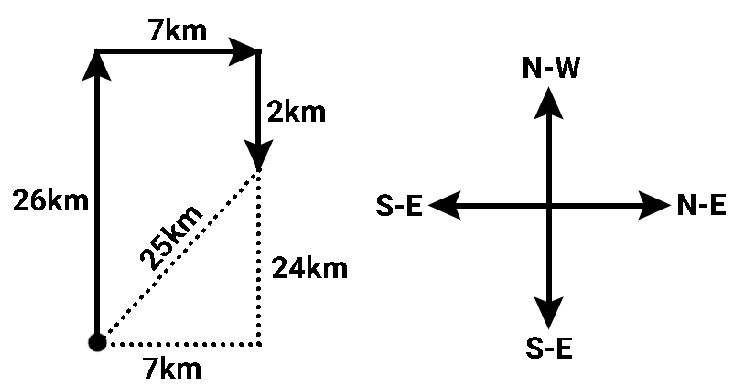Here, the required distance is the hypotenuse of the triangle with the base as 7 km and height as 24km (26-2).
The smallest distance is shown by the dotted line which is $\sqrt{7^2 + 24^2} =25$ km
Hence, 25km is the correct answer

The path travelled is as shown in the figure.If the initial direction is west, then the final direction is south as shown.

Ajay starts from his home and heads east. He walks for 3 km and then takes a left turn. After walking for another 2 km, he takes a right turn. He walks for another 3 km and then takes another left. He walks for 2 more km before coming to a halt. We can represent this situation in the form of the following figure.The shortest distance between H and F will be given by
$6^2 + 4^2 = \sqrt{52} = 2\sqrt{13}$
Hence the correct answer is option B.

The person proceeds 15 m in West Direction.
Turning left means, he would be facing South.
He moves 15 m in South Direction.
Therefore, the person is in South-West direction of the initial position
Hence, the Option B is the answer

The points A, B and C are as shown in the figure below.The points A, B and C form a right angled triangle.
Applying Pythagoras theorem we get,
$AC^2=AB^2+BC^2$
$=>AC^2=8^2+15^2$
$=>AC^2=289$
$=>AC=17$ km

The following figure illustrates his trajectory-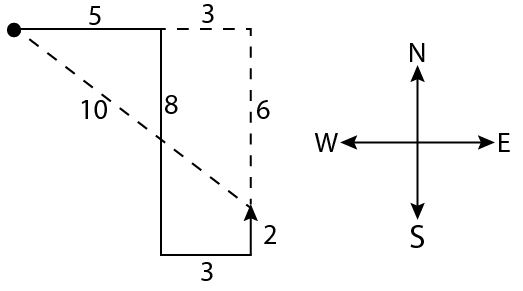Thus, he is finally facing the North Direction and is $\sqrt{8^2 + 6^2} = 10$ km away from his home.

Based on the given information, the following diagram could be derive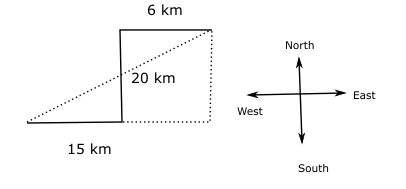55The distance between starting and ending points = $\sqrt{20^2 + (15+6)^2}$ = 29 km.

Lohit’s path can be drawn as shown in the figure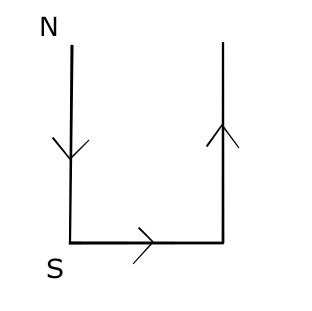Initially, he is facing the north and walking towards the south.
Then, he takes a right and walks east facing east.
Then, he takes a right and faces the south and walks towards the north.
Hence, B is the correct answer.

Mohan starts from his home and heads north. He walks for 3 km and then takes a left turn. He walks for another 2 km and then takes a right turn. He walks for 1 km and then takes a left turn. He walks for another 1 km
We can represent this information in the following diagram.Hence the shortest distance between the initial and final positions can be obtained by using Pythagoras theorem. This distance is given by
d = $\sqrt{4^2 + 3^2} = \sqrt{25} = 5 km$
Hence option C is the correct answer.

Pankaj starts from his home and heads west. He walks for 4 km and then takes a right turn. He walks for another 3 km and then takes a left turn. He walks for another 2 km and then takes a right turn. He stops after walking for another 3 km. This situation can be represented through the following diagram.The shortest distance between his initial and final positions can be obtained by using the Pythagoras theorem. This, distance is given by
D = $\sqrt{6^2 + 6^2} = 6\sqrt{2}$
Thus, option C is the correct answer.

The path can be drawn as below.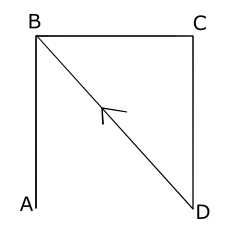Hence point B is to the North-west of point D.

Let North direction be ‘j’, South direction be ‘-j’ , West direction be ‘-I’ and East direction be ‘i’ .
=> 4(j) + 5(-i) + 5(-j) + 6(i) + 1(j)
=> 1(i)
Since he is one unit East of the initial point, he would have to go in West Direction
Hence, correct option is B

The question only asks for the final direction.
So the distances travelled need not be considered.
The person travelling takes one left and then one right.
By doing so his final direction will be the same as his initial direction.
Hence the final direction is south.

Let Abhay started from point A and travelled north for 9 km and reached B, then turned left towards west and traveled 5 km, then again turned left and finally stopped at point D after travelling 9 km.Thus, AD = BC = 5 km$\therefore$ He is 5 km to the west of his starting point.=> Ans – (A)

Let Sunita started from point A, and travelled north to reach B, then she turned left and reached point C, again she turned left and travelled for 4 km. So, D is 2 km to the west of A.Thus, AB = CD = 4 km=> Ans – (C)

Let Pinky started from point A and travelled 600 m east to reach B, then she turned left to reach C after travelling 500 m, again she turned left and travelled for 600 m, and finally stopped at point A.$\because$ Her final position and initial positions are same, thus she is 0 m from the starting point.=> Ans – (D)

Let Anand started from point A and travelled east for 10 km to reach his school at B, then travelled south for 5 km to reach his father’s shop at C, finally travelled west for 10 km to stop at point D.Thus, AD = 5 km$\therefore$ Anand is 5 km to the south of his home.=> Ans – (B)